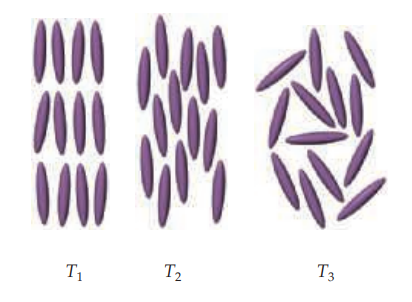×
Get Full Access to Chemistry: The Central Science - 14 Edition - Chapter 11 - Problem 11.8
Get Full Access to Chemistry: The Central Science - 14 Edition - Chapter 11 - Problem 11.8

×

# ?At three different temperatures, $$T_{1}, T_{2}, \text { and } T_{3}$$, the molecules in a liquid crystal align in these ways:ISBN: 9780134414232 1274

## Solution for problem 11.8 Chapter 11

Chemistry: The Central Science | 14th Edition

• Textbook Solutions
• 2901 Step-by-step solutions solved by professors and subject experts
• Get 24/7 help from StudySoup virtual teaching assistantsChemistry: The Central Science | 14th Edition

4 5 1 259 Reviews
18
1
Problem 11.8

At three different temperatures, $$T_{1}, T_{2}, \text { and } T_{3}$$, the molecules in a liquid crystal align in these ways:(a) At which temperature or temperatures is the substance in a liquid crystalline state? At those temperatures, which type of liquid crystalline phase is depicted?

(b) Which is the highest of these three temperatures? [Section 11.7]

Text Transcription:

T_1, T_2,and  T_3

Step-by-Step Solution:

Step 1 of 5) If we can measure the equilibrium concentrations of all the reactants and products in a chemical reaction, as we did with the data in Table 15.1, calculating the value of the equilibrium constant is straightforward. We simply insert all the equilibrium concentrations into the equilibrium-constant expression for the reaction.We are given a balanced equation and equilibrium partial pressures and are asked to calculate the value of the equilibrium constant. Plan Using the balanced equation, we write the equilibrium constant expression. We then substitute the equilibrium partial pressures into the expression and solve for Kp.We tabulate the initial and equilibrium concentrations of as many species as we can. We also provide space in our table for listing the changes in concentrations. As shown, it is convenient to use the chemical equation as the heading for the table.We calculate the change in HI concentration, which is the difference between the equilibrium and initial values:

Step 2 of 2

##### ISBN: 9780134414232

This textbook survival guide was created for the textbook: Chemistry: The Central Science, edition: 14. Chemistry: The Central Science was written by and is associated to the ISBN: 9780134414232. Since the solution to 11.8 from 11 chapter was answered, more than 219 students have viewed the full step-by-step answer. This full solution covers the following key subjects: . This expansive textbook survival guide covers 29 chapters, and 2820 solutions. The full step-by-step solution to problem: 11.8 from chapter: 11 was answered by , our top Chemistry solution expert on 10/03/18, 06:29PM. The answer to “?At three different temperatures, $$T_{1}, T_{2}, \text { and } T_{3}$$, the molecules in a liquid crystal align in these ways: (a) At which temperature or temperatures is the substance in a liquid crystalline state? At those temperatures, which type of liquid crystalline phase is depicted? (b) Which is the highest of these three temperatures? [Section 11.7]Text Transcription:T_1, T_2,and T_3” is broken down into a number of easy to follow steps, and 60 words.

## Discover and learn what students are asking

Calculus: Early Transcendental Functions : Conics, Parametric Equations, and Polar Coordinates
?In Exercises 1–6, match the equation with the correct graph. [The graphs are labeled (a), (b), (c), (d), (e), and (f).]

Unlock Textbook Solution

?At three different temperatures, $$T_{1}, T_{2}, \text { and } T_{3}$$, the molecules in a liquid crystal align in these ways: